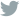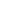# A Recurrent Latent Variable Model for Sequential Data

27 April 2018

by Saleem Ahmedtags: rnn variational autoencoder deep-learning NIPS2015

### The inclusion of latent random variables into the hidden state of a recurrent neural network (RNN) by combining the elements of the variational autoencoder. Use of high-level latent random variables of the variational RNN (VRNN) to model the kind of variability observed in highly structured sequential data such as natural speech.

Target: Learning generative models of sequences.

**Argument: **Complex dependencies cannot be modelled efficiently by the output probability models used in standard RNNs, which include either a simple unimodal distribution or a mixture of unimodal distributions.

**Assumptions: **Only concerned with highly structured data having -

• relatively high signal-to-noise ratio, variability observed is due to signal not noise.

• underlying factors of variation and the observed data are strongly correlated.

## Why is the architecture so powerful and how it is differentiated by other methods?

Hypothesis : Model variability should induce temporal dependencies across timesteps.

**Implement : **Like DBN models such as HMMs and Kalman filters, model the dependencies between the latent random variables across timesteps.

Innovation : Not the first to propose integrating random variables into the RNN hidden state, the first to integrate the dependencies between the latent random variables at neighboring timesteps. Extend the VAE into a recurrent framework for modelling high-dimensional sequences.

## **What implementation **is available? (Setting up the code environment)

Most of the script files are written as pure Theano code, modules are implemented from a more general framework written by original author, Junyoung Chung.

Yoshua Bengio announced on Sept. 28, 2017, that development on Theano would cease. Theano is effectively dead.

Many academic researchers in the field of deep learning rely on Theano, the grand-daddy of deep-learning frameworks, which is written in Python. Theano is a library that handles multidimensional arrays, like Numpy. Used with other libs, it is well suited to data exploration and intended for research.

Numerous open-source deep-libraries have been built on top of Theano, including Keras, Lasagne and Blocks. These libs attempt to layer an easier to use API on top of Theano’s occasionally non-intuitive interface. (As of March 2016, another Theano-related library, Pylearn2, appears to be dead.)

Pros and Cons

• (+) Python + Numpy

• (+) Computational graph is nice abstraction

• (+) RNNs fit nicely in computational graph

• (-) Raw Theano is somewhat low-level

• (+) High level wrappers (Keras, Lasagne) ease the pain

• (-) Error messages can be unhelpful

• (-) Large models can have long compile times

• (-) Much “fatter” than Torch

• (-) Patchy support for pretrained models

• (-) Buggy on AWS

• (-) Single GPU

## What can you improve? (Your contribution to the existing code.)

Possible ideas :

• Implementation in an entirely new framework like pytorch/tensorflow which is much more gpu friendly thus allowing for testing on larger more higher dimensional datasets. Like probable chess moves.

• The VRNN contains a VAE at every timestep. However, these VAEs are conditioned on the state variable ht − 1 of an RNN. This addition will help the VAE to take into account the temporal structure of the sequential data. Experimenting with a replay buffer and batch normalizations could probably have a huge impact.

• More efficient use of previous experience, by learning with it multiple times. This is key when gaining real-world experience is costly, you can get full use of it. The memory-learning updates are incremental and do not converge quickly, so multiple passes with the same data is beneficial, especially when there is low variance in immediate outcomes (reward, next state) given the same state, action pair.

• Better convergence behaviour when training a function approximator. Partly this is because the data is more like i.i.d. data assumed in most supervised learning convergence proofs.

• It is harder to use multi-step learning algorithms, such as Q(λ), which can be tuned to give better learning curves by balancing between bias (due to bootstrapping) and variance (due to delays and randomness in long-term outcomes)

## Describe the Dataset in use. Can you apply these methods to some other dataset?

Comparison : The proposed VRNN model against other RNN-based models – including a VRNNmodel without introducing temporal dependencies between the latent random variables.

• Modelling natural speech directly from the raw audio waveforms

• Modelling handwriting generation.

Speech Modelling : Directly model raw audio signals, represented as a sequence of 200-dimensional frames. Each frame corresponds to the real-valued amplitudes of 200 consecutive raw acoustic samples. Note that this is unlike the conventional approach for modelling speech, often used in speech synthesis where models are expressed over representations such as spectral features.

Evaluation -

• Blizzard: This text-to-speech dataset made available by the Blizzard Challenge 2013 contains 300 hours of English, spoken by a single female speaker.

• TIMIT: This widely used dataset for benchmarking speech recognition systems contains 6300 English sentences, read by 630 speakers.

• Onomatopoeia2 : This is a set of 6, 738 non-linguistic human-made sounds such as coughing, screaming, laughing and shouting, recorded from 51 voice actors.

• Accent: This dataset contains English paragraphs read by 2, 046 different native and nonnative English speakers.

**Handwriting Modelling : **Each model learn a sequence of (x, y) coordinates together with binary indicators of pen-up/pen-down.

**Evaluation - **

• IAM-OnDB: dataset which consists of 13040 handwritten lines written by 500 writers.

Changes on this project could potentially work on any generic sequential datasets.

Example :

• Sequential penstroke data of MNIST : The MNIST handwritten digit images transformed into a data set for sequence learning. This data set contains pen stroke sequences based on the original MNIST images.

• The most popular benchmarks for sequential data are PTB and TIMIT, authors used TIMIT but not PTB.

Implementation based on Chung’s A Recurrent Latent Variable Model for Sequential Data [arXiv:1506.02216v6].

### 1. Network design

There are three types of layers: input (x), hidden(h) and latent(z). We can compare VRNN sided by side with RNN to see how it works in generation phase.

• RNN: $h_o + x_o -> h_1 + x_1 -> h_2 + x_2 -> …$
• VRNN: with $h_o \left{ \begin{array}{ll} h_o -> z_1 z_1 + h_o -> x_1 z_1 + x_1 + h_o -> h_1 \end{array} \right .$ with $h_1 \left{ \begin{array}{ll} h_1 -> z_2 z_2 + h_1 -> x_2 z_2 + x_2 + h_1 -> h_2 \end{array} \right .$

It is clearer to see how it works in the code blocks below. This loop is used to generate new text when the network is properly trained. x is wanted output, h is deterministic hidden state, and z is latent state (stochastic hidden state). Both h and z are changing with repect to time.

### 2. Training

The VRNN above contains three components, a latent layer genreator $h_o -> z_1$, a decoder net to get $x_1$, and a recurrent net to get $h_1$ for the next cycle.

The training objective is to make sure $x_0$ is realistic. To do that, an encoder layer is added to transform $x_1 + h_0 -> z_1$. Then the decoder should transform $z_1 + h_o -> x_1$ correctly. This implies a cross-entropy loss in the “tiny shakespear” or MSE in image reconstruction.

Another loose end is $h_o -> z_1$. Statistically, $x_1 + h_0 -> z_1$ should be the same as $h_o -> z_1$, if $x_1$ is sampled randomly. This constraint is formularize as a KL divergence between the two.

#### KL Divergence of Multivariate Normal DistributionNow putting everything together for one training cycle.

$\left{ \begin{array}{ll} h_o -> z_{1,prior} x_1 + h_o -> z_{1,infer} z_1 <- sampling N(z_{1,infer}) z_1 + h_o -> x_{1,reconstruct} z_1 + x_1 + h_o -> h_1 \end{array} \right .$ => $\left{ \begin{array}{ll} loss_latent = DL(z_{1,infer} | z_{1,prior}) loss_reconstruct = x_1 - x_{1,reconstruct} \end{array} \right .$

Pytorch implementation of the Variational RNN (VRNN), from A Recurrent Latent Variable Model for Sequential Data.

The paper is available here.## Run:

To train: python train.py

To sample with saved model: python sample.py [saves/saved_state_dict_name.pth]

back